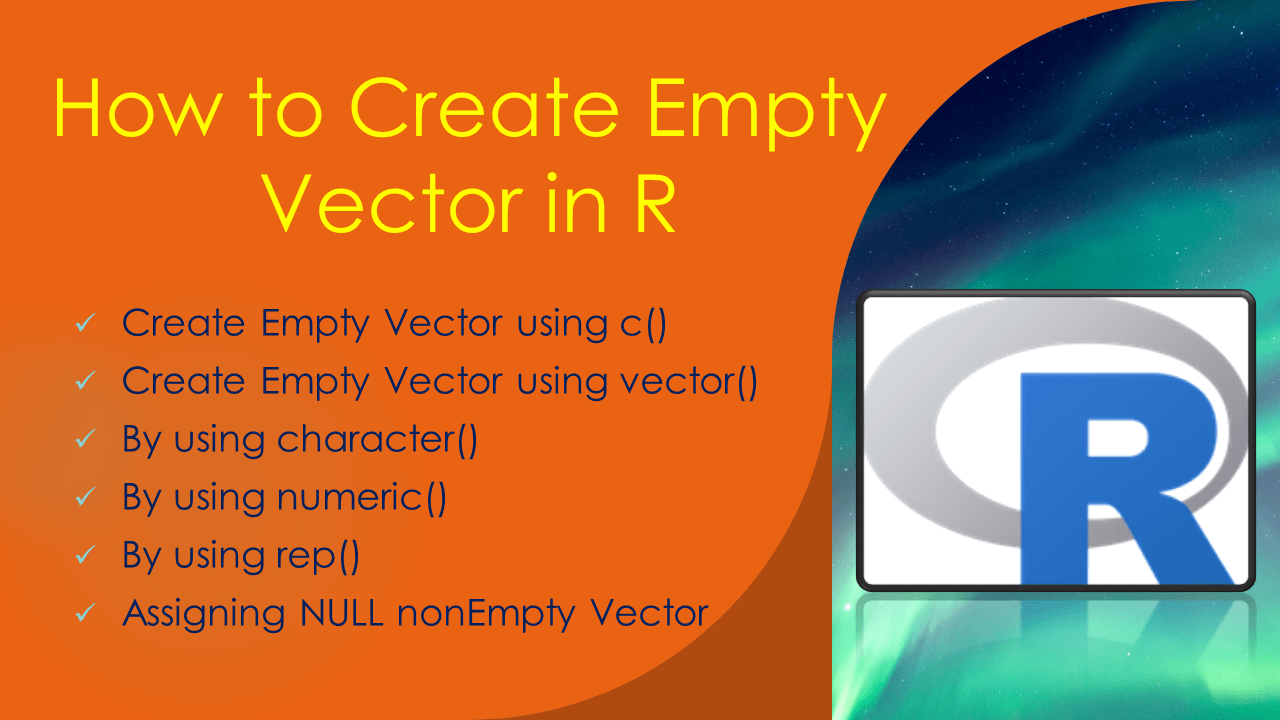# How to Create Empty Vector in R

There are several ways to create an empty vector in R. If a vector contains zero length with out any elements is considered an empty vector. A vector in R is a basic data structure that contains elements of the same type.

The following methods are used to create an empty vector in R, I will explain all these with examples.

• c()
• vector()
• character()
• numeric()
• rep()

Though all these methods are used to create an empty vector, each method stores different value for empty and different data type. Use `is.null()` function to check if the vector is NULL or not. The `is.null()` function returns a boolean vector that is either TRUE or FALSE.

## 1. Quick Examples of Create Empty Vector

The follwoing are quick examples of create empty vector in R.

``````
# Quick Examples

# Create empty vector using c()
v <- c()
print(v)
length(v)
typeof(v)

# Create Empty vector using vector()
v <- vector()
print(v)
typeof(v)

# Create empty vector of type character
v <- character()
print(v)
typeof(v)

# Create empty vector of type double
v <- numeric()
print(v)
typeof(v)

# Using rep() function
v <- rep(NULL, 0)
print(v)
length(v)
``````

## 2. Create Empty Vector using c()

One of the most used way to create a vector in R is by using c() combined function. Use this c() function with out any arguments to initialize an empty vector.

``````
# Create empty vector using c()
v <- c()
print(v)

# Output
#> print(v)
#NULL
``````

Use `length()` function to get the length of the vector. For empty vector the length would be 0.

``````
# Get length of empty vector
length(v)

# Output
#> length(v)
# 0
``````

The empty vector created with `c()` returns `NULL` as type of the vector.

``````
# Type of vector
typeof(v)

# Output
#> typeof(v)
# "NULL"
``````

In case you wanted to add element to a vector use the assigned operator or an `append()` function. In the below example I have added A and B values to the vector. After inserting the character elements note the type is changed to character vector.

``````
# Add element to empty Vector
v <- append(v,c('A','B'))
print(v)
typeof(v)

# Output
#> print(v)
# "A" "B"
#> typeof(v)
# "character"
``````

## 3. Create Empty Vector using vector()

The `vector()` function can also be used to create an empty vector in R, by default it creates logical type. vector() function takes syntax `vector(mode = "logical", length = 0)` so to initialize an empty vector you don’t have to use any arguments. Note that printing empty vector using `print(v)` displays `logical(0)`.

``````
# Create Empty Vector
v <- vector()
print(v)
typeof(v)

# Output
#> print(v)
#logical(0)
#> typeof(v)
# "logical"
``````

## 4. By using character()

`character()` function in R is used to create a character vector. By default this function initializes a vector of specified length with all empty string as values, so to create empty vector just use `character()` function with out any arguments. It creates a vector of type `character`.

``````
# Create empty vector of type character
v <- character()
print(v)
typeof(v)

# Output
#> print(v)
#character(0)
#> typeof(v)
# "character"
``````

## 5. By using numeric()

The `numeric()` function in R creates a double-precision vector of the length specified in the argument with all values zero. It create a vector of type `numeric`.

``````
# Create empty vector of type double
v <- numeric()
print(v)
typeof(v)

# Output
#> print(v)
#numeric(0)
#> typeof(v)
# "double"
``````

## 6. By using rep()

The `rep()` is a generic function that is used to replicate the values in the input data.

``````
# Using rep() function
v <- rep(NULL, 0)
print(v)
length(v)

# Output
#> print(v)
#NULL
#> length(v)
# 0
``````

## 7. Assigning NULL non Empty Vector

Finally, you can also create an empty vector in R by assigning a NULL value to exsisting vector. By doing this we are converting non empty vector to empty vector.

``````
# By Assigning NULL
v <- c('A','B')
v <- NULL
print(v)
length(v)
typeof(v)

# Output
> print(v)
NULL
> length(v)
 0
> typeof(v)
 "NULL"
``````

Note that `is.null()` function returns `FALSE` for vector(), character() and c() functions and `TRUE` for rest of the methods.

## Conclusion

In this aricle, you have learned how to create an empty vector by using c(), vector(), character(), rep() functions. You can find the complete example from this article at Github R Programming Examples Project.

## Related Articles

### Naveen (NNK)

Naveen (NNK) is a Data Engineer with 20+ years of experience in transforming data into actionable insights. Over the years, He has honed his expertise in designing, implementing, and maintaining data pipelines with frameworks like Apache Spark, PySpark, Pandas, R, Hive and Machine Learning. Naveen journey in the field of data engineering has been a continuous learning, innovation, and a strong commitment to data integrity. In this blog, he shares his experiences with the data as he come across. Follow Naveen @ @ LinkedIn